Next: 5. Conclusions, some final Up: 4. Fractal Strings Previous: 4.1 The Hausdorf dimension

## 4.2 Classical-to-fractal geometric transition

The role of area resolution, as pointed out in the previous sections, leads us to search for a critical area characterizing the transition from classical to fractal geometry of the string stack. To this end, we consider tha case in which the string possesses a non vanishing average momentum, in which case we may use a gaussian wave packet of the form (27). The corresponding wave functional, in the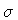-representation, is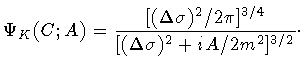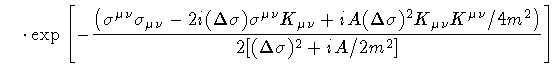(44)

where we have used equation (34) to exchange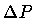with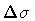. The corresponding probability density evolves'' as follows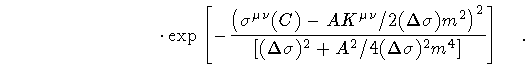(45)

Therefore, the average area variation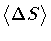, when the loop wave packet drifts with a momentum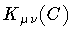, is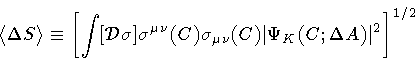(46)

For our purpose, there is no need to compute the exact form of the mean value (46), but only its dependence on. This can be done in three steps:
1.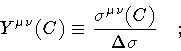(47)

2.
shift the new integration variable as follows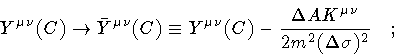(48)

3.
rescale the integration variable as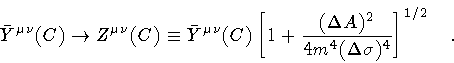(49)

Then, we obtain=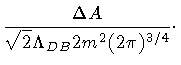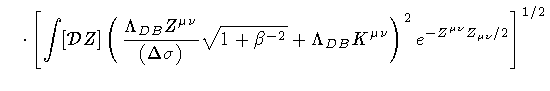(50)

where,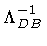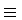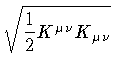(51)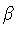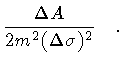(52)

The parametermeasures the ratio of the temporal'' to spatial'' uncertainty, while the areasets the scale of the surface variation at which the string momentum is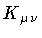. Therefore, always with the particle analogy in mind, we shall callthe loop DeBroglie area. Let us assume, for the moment, that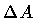is independent of, so that either quantity can be treated as a free parameter in the theory. A notable exception to this hypothesis will be discussed shortly. Presently, we note that taking the limit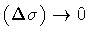, affects only the first term of the integral (50), and that its weight with respect to the second term is measured by the ratio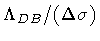. If the area resolution is much larger than the loop DeBroglie area, then the first term is negligible:is independent ofand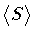scales as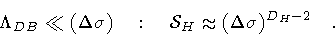(53)

In this case, independence ofis achieved by assigning D H = 2. As one might have anticipated, the detecting apparatus is unable to resolve the graininess of the string stack, which therefore appears as a smooth two dimensional surface.
The fractal, or quantum, behavior manifests itself below, when the first term in (50) provides the leading contribution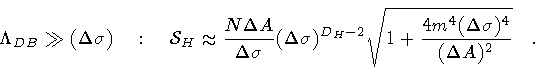(54)

This expression is less transparent than the relation (53), as it involves also theresolution. However, one may now consider two special subcases in which the Hausdorff dimension can be assigned a definite value.
In the first case, we keepfixed, and scaledown to zero. Then, each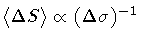diverges, because of larger and larger shape fluctuations, and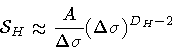(55)

requires D H = 3.
The same result can be obtained also in the second subcase, in which bothandscale down to zero, but in such a way that their ratio remains constant,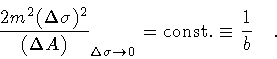(56)

The total interior area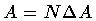is kept fixed. Therefore, as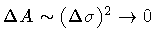, thenin order to keep A finite. Then,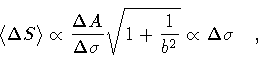(57)

and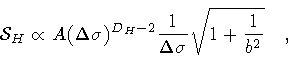(58)

which leads to D H = 3 again. In the language of fractal geometry, this interesting subcase corresponds to self-similarity. Thus, the condition (57) defines a special class of self-similar loops characterized by an average area variation which is proportional toat any scale.Next: 5. Conclusions, some final Up: 4. Fractal Strings Previous: 4.1 The Hausdorf dimension